﻿ 中国跨越“中等收入陷阱”的路径突破——基于增长收敛理论的识别及“双轮驱动”检验
 上海财经大学学报2018, Vol. 20Issue (1): 29-420

#### 文章信息上海财经大学2018年20卷第1期

Liu Jinquan, Wang Qiaoru, Liu Dayu

The Path Breakthrough of Escaping the “Middle-income Trap” in China: Based on an Identification Method of Growth Convergence Theory and “Two-wheel Drive” Test

Journal of Shanghai University of Finance and Economics, 2018, 20(1): 29-42.

### 文章历史《上海财经大学学报》
2018第20卷第1期

 $Y\left( t \right) = F\left( {K\left( t \right),A\left( t \right)L\left( t \right)} \right)$ (1)

 $k\left( t \right) = \frac{{K\left( t \right)}}{{A\left( t \right)L\left( t \right)}},\;f\left( {k\left( t \right)} \right){\rm{ = }}\frac{{Y\left( t \right)}}{{A\left( t \right)L\left( t \right)}} = F\left( {\frac{{K\left( t \right)}}{{A\left( t \right)L\left( t \right)}},1} \right)$ (2)

 $y\left( t \right) = \frac{{Y\left( t \right)}}{{L\left( t \right)}}$ (3)

 $\frac{{\dot k\left( t \right)}}{{k\left( t \right)}} = \frac{{sf\left( {k\left( t \right)} \right)}}{{k\left( t \right)}} - \left( {\delta + a + n} \right)$ (4)

 $\dot k\left( t \right) = sf\left( {k\left( t \right)} \right) - \left( {\delta + a + n} \right)k\left( t \right)$ (5)

 $sf\left( {{k^*}} \right) - \left( {\delta + a + n} \right){k^*} = 0$ (6)

 $g = \frac{{\dot y\left( t \right)}}{{y\left( t \right)}} = a + \varepsilon \left( k \right)\frac{{\dot k\left( t \right)}}{{k\left( t \right)}}$ (7)

 $g \simeq \frac{{\dot y\left( t \right)}}{{y\left( t \right)}} \approx a - \varepsilon \left( {{k^*}} \right)\left( {1 - \varepsilon \left( {{k^*}} \right)} \right)\left( {\delta + a + n} \right)\left( {\ln k\left( t \right) - \ln {k^*}} \right)$ (8)

 $\ln y\left( t \right) - \ln {y^*}\left( t \right) \simeq \varepsilon \left( {{k^*}} \right)\left( {\ln k\left( t \right) - \ln {k^*}} \right)$ (9)

 $g = \frac{{\dot y\left( t \right)}}{{y\left( t \right)}} \simeq a - \left( {1 - \varepsilon \left( {{k^*}} \right)} \right)\left( {\delta + a + n} \right)\left( {\ln y\left( t \right) - \ln {y^*}\left( t \right)} \right)$ (10)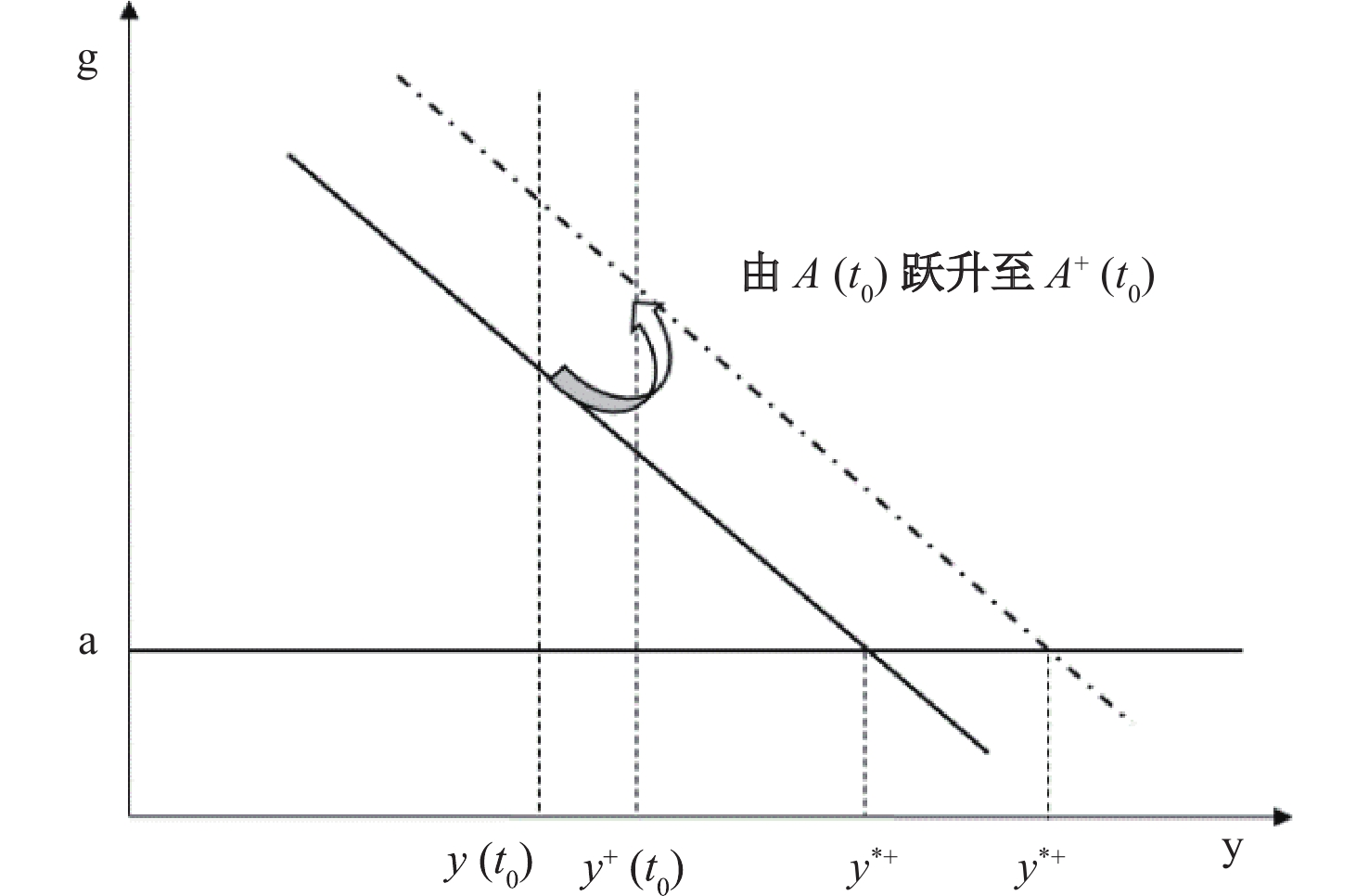图 1 收敛路径的转变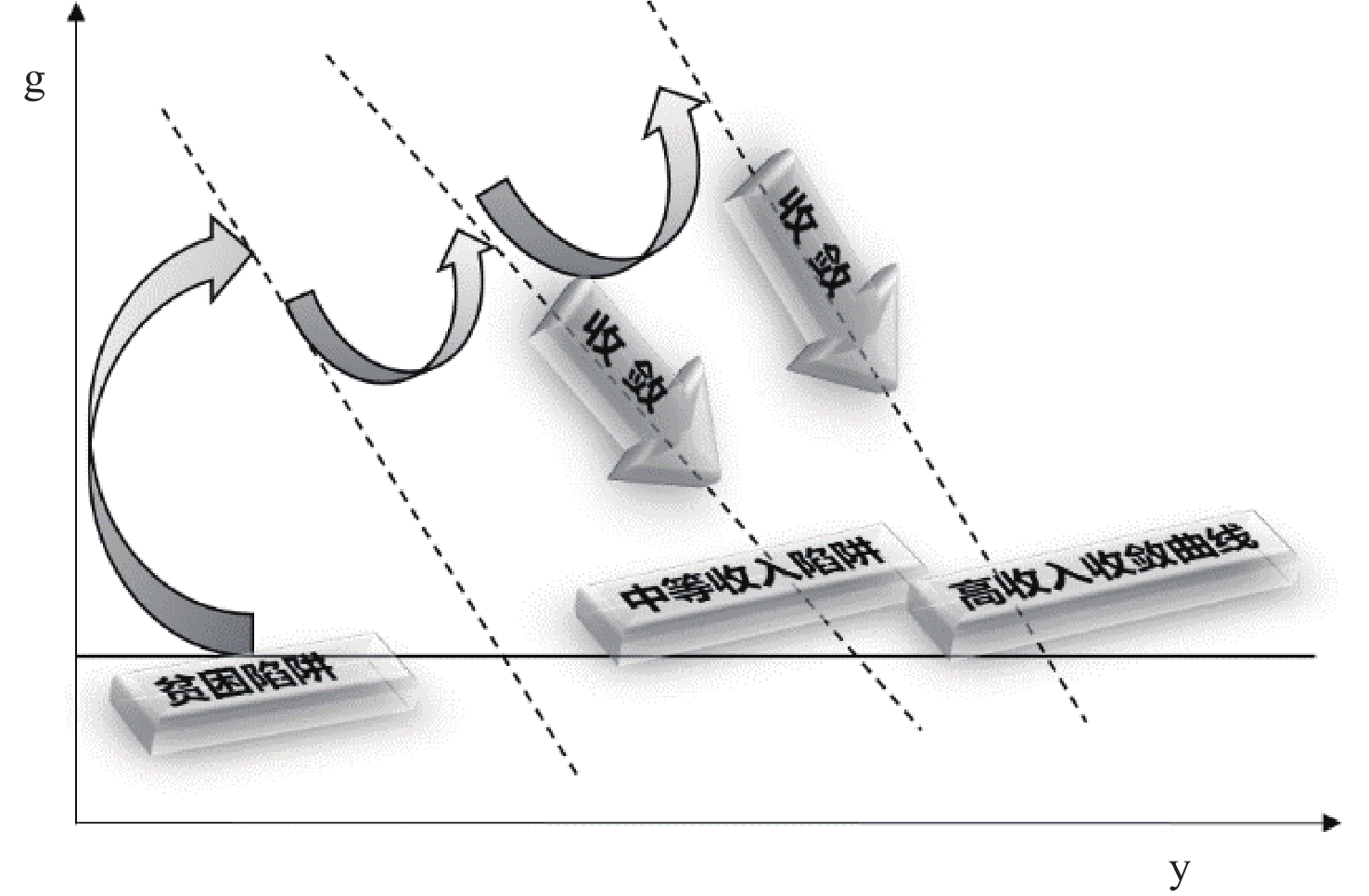图 2 经济增长收敛模式

 ${g_t} = \alpha + \beta \left[ {\ln {y_t} - \ln y_t^*} \right] + {\varepsilon _t}$ (11)

 ${g_t} = (\alpha + \beta {\rm{gap}})I\left( {gap {\text{≤}} {\gamma _1}} \right) + (\alpha ' + \beta '{\rm{gap}})I\left( {{\gamma _1} < gap {\text{≤}} {\gamma _2}} \right) + (\alpha '' + \beta ''{\rm{gap}})I\left( {gap > {\gamma _2}} \right) + {\mu _t}$ (12)

(二) 门限回归模型在收敛路径识别与检验上的应用

 $F = T\left( {{{\tilde \sigma }^2} - {{\hat \sigma }^2}} \right)/{\hat \sigma ^2}$ (13)

 $F = \mathop {\sup }\limits_{{{\hat \gamma }_ * } \in \Gamma } \left( {F\left( {{{\hat \gamma }_ * }} \right)} \right)$ (14)
 $F\left( {{\gamma _ * }} \right) = T\left( {{{\tilde \sigma }^2} - {{\hat \sigma }^2}\left( \gamma \right)} \right)/{\hat \sigma ^2}\left( \gamma \right)$ (15)

 $W\left( {{\gamma _ * }} \right) = {\left[ {R\hat \beta \left( {{\gamma _ * }} \right)} \right]^\prime }{\left[ {R\left( {M{{\left( {{\gamma _ * }} \right)}^{ - 1}}V\left( {{\gamma _ * }} \right)M{{\left( {{\gamma _ * }} \right)}^{ - 1}}} \right)R'} \right]^{ - 1}}R\hat \beta \left( {{\gamma _ * }} \right)$ (16)
 $M\left( {{\gamma _ * }} \right) = \sum\nolimits_{t = 1}^T {{x_t}\left( {{\gamma _ * }} \right)} {x_t}^\prime \left( {{\gamma _ * }} \right),\;V\left( {{\gamma _ * }} \right) = \sum\nolimits_{t = 1}^T {{x_t}\left( {{\gamma _ * }} \right)} {x_t}^\prime \left( {{\gamma _ * }} \right)\hat \varepsilon _t^2$ (17)

 $W = \mathop {\sup }\limits_{{{\hat \gamma }_ * } \in \Gamma } \left( {F\left( {{{\hat \gamma }_ * }} \right)} \right)$ (18)

 线性方程 区制1 区制2 区制3 参数 估计值 标准差 参数 估计值 标准差 参数 估计值 标准差 参数 估计值 标准差 ${ \bar \alpha }$ 5.252*** 0.611 α –21.849*** 0.923 α' 37.979*** 1.500 α'' –1.832* 0.949 ${ \,\bar \beta }$ –1.237*** 0.206 β –8.168*** 0.235 β' 8.445*** 0.428 β'' –4.466*** 0.469 R2 0.198 R2 0.998 R2 0.965 R2 0.701 门限 — 门限 ${ gap {\text{≤}} - 3.688}$ 门限 ${ - 3.688 < {\rm{g}}ap {\text{≤}} - 2.793}$ 门限 ${ gap > - 2.793}$ 门限效应P值：— 门限效应P值：0.000 门限效应P值：0.000 门限效应P值：0.000 注：括号内为对应的标准误差，***、**与*分别代表1%、5%与10%的显著性水平。

(一) LT-TVP-VAR模型结构设定

 $A{y_t} = {F_1}{y_{t - 1}} + \cdots + {F_s}{y_{t - s}} + {\mu _t},\;\;t = s + 1, \cdots ,n$ (19)

 $\Sigma = \left[ {\begin{array}{*{20}{c}}{{\sigma _1}}&0& \cdots &0\\0&{{\sigma _2}}& \ddots &0\\ \vdots & \ddots & \ddots & \vdots \\[4pt]0&0& \cdots &{{\sigma _k}}\end{array}} \right]\;\;\;\;\;\;A = \left[ {\begin{array}{*{20}{c}}1&0& \cdots &0\\{{a_{2,1}}}& \ddots & \ddots &0\\ \vdots & \ddots & \ddots & \vdots \\[4pt]{{a_{k,1}}}& \cdots &{{a_{k,k - 1}}}&1\end{array}} \right]$

${\beta _i} = {A^{ - 1}}{F_i}$ ${X_t} = {I_s} \otimes \left( {{y_{t - 1}}, \cdots ,{y_{t - s}}} \right)$ $\otimes$ 为克罗内克积，将时变参数引入后，方程（19）可简化为如下形式：

 ${y_t} = {X_t}{\beta _t} + A_t^{ - 1}{\Sigma _t}{\varepsilon _t},\;\;t = s + 1, \cdots ,n$ (20)

 \begin{aligned}\begin{aligned}{\beta _t} = {\mu _\beta } + {\Phi _\beta }\left( {{\beta _{t - 1}} - {\mu _\beta }} \right) + {v_t},t = s + 1, \cdots ,n,\\{\alpha _t} = {\mu _\alpha } + {\Phi _\alpha }\left( {{\alpha _{t - 1}} - {\mu _\alpha }} \right) + {\zeta _t},t = s + 1, \cdots ,n,\\{h_t} = {\mu _h} + {\Phi _h}\left( {{h_{t - 1}} - {\mu _h}} \right) + {\xi _t},t = s + 1, \cdots ,n,\end{aligned}&\;\;\;{V = Var\left( {\begin{aligned}{{\varepsilon _t}}\\{{v_t}}\\{{\zeta _t}}\\{{\xi _t}}\end{aligned}} \right) = \left( {\begin{aligned}& {{I_n}}\;\;\;0\;\;\;\;\;\;\;0\;\;\;\;0\\& 0\;\;\;\;{{\Omega _\beta }}\;\;\;\;0\;\;\;\;0\\& 0\;\;\;\;0\;\;\;\;\;\;{{\Omega _\alpha }}\;\;0\\& 0\;\;\;\;0\;\;\;\;\;\;\;0\;\;\;\;{{\Omega _h}}\end{aligned}} \right)}\end{aligned} (21)

 ${b_t} = {\beta _t} \times I\left( {\left| {{\beta _t}} \right| {\text{≥}} {d_b}} \right)$ (22)
 ${a_t} = {\alpha _t} \times I\left( {\left| {{\alpha _t}} \right| {\text{≥}} {d_a}} \right)$ (23)

(二) 数据处理与估计

 参数 均值 标准差 无效影响因子 ${ {\mu _\beta }}$ 0.029 2 0.017 1 34.74 ${{\Phi _\beta }}$ 0.808 9 0.081 0 72.88 ${{\left( {{\Omega _\beta }} \right)_1}}$ 0.021 2 0.001 9 83.53 ${{\mu _\alpha }}$ –0.1764 0.157 8 59.46 ${{\Phi _\alpha }}$ 0.914 4 0.058 6 66.66 ${{\left( {{\Omega _\alpha }} \right)_1}}$ 0.075 5 0.022 4 103.32 ${{\mu _h}}$ 0.017 0 0.017 6 90.76 ${{\Phi _h}}$ 0.878 4 0.060 2 57.01 ${{\left( {{\Omega _h}} \right)_1}}$ 1.170 8 0.361 2 214.54 ${{\left( {{d_b}} \right)_1}}$ 0.043 6 0.039 2 163.96 ${{\left( {{d_b}} \right)_2}}$ 0.187 8 0.108 1 5.04 ${{\left( {{d_a}} \right)_1}}$ 0.178 1 0.109 7 101.74 ${{\left( {{d_a}} \right)_2}}$ 0.354 7 0.354 0 170.34

 参数 ${{\left( {{d_b}} \right)_1}}$ ${{\left( {{d_b}} \right)_2}}$ ${{\left( {{d_a}} \right)_1}}$ ${{\left( {{d_a}} \right)_2}}$ 可接受率 43.3 24.1 89.0 48.3
(三) 经济增长动力机制的时变特征分析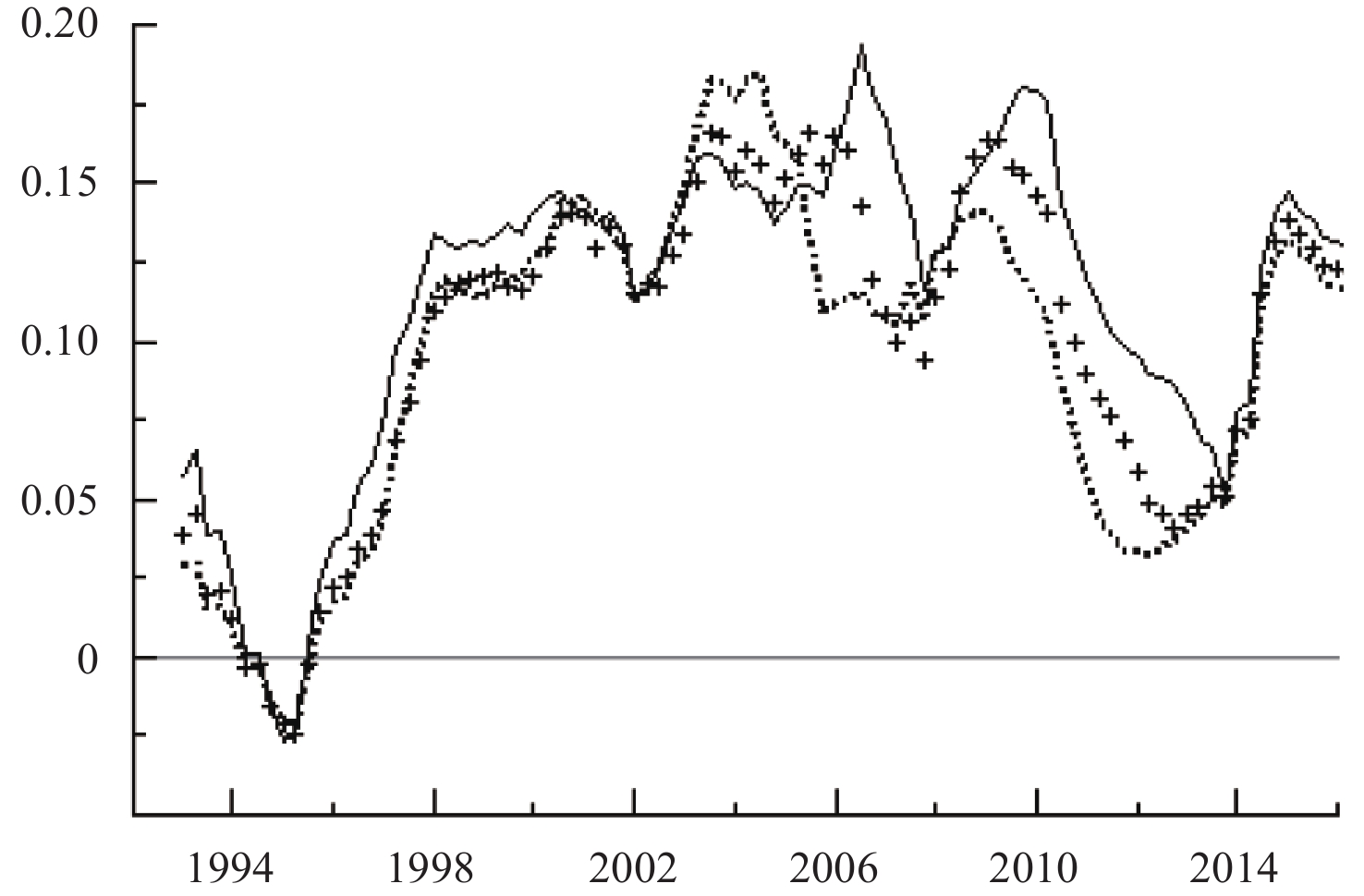图 3 技术进步对经济增长冲击的时变响应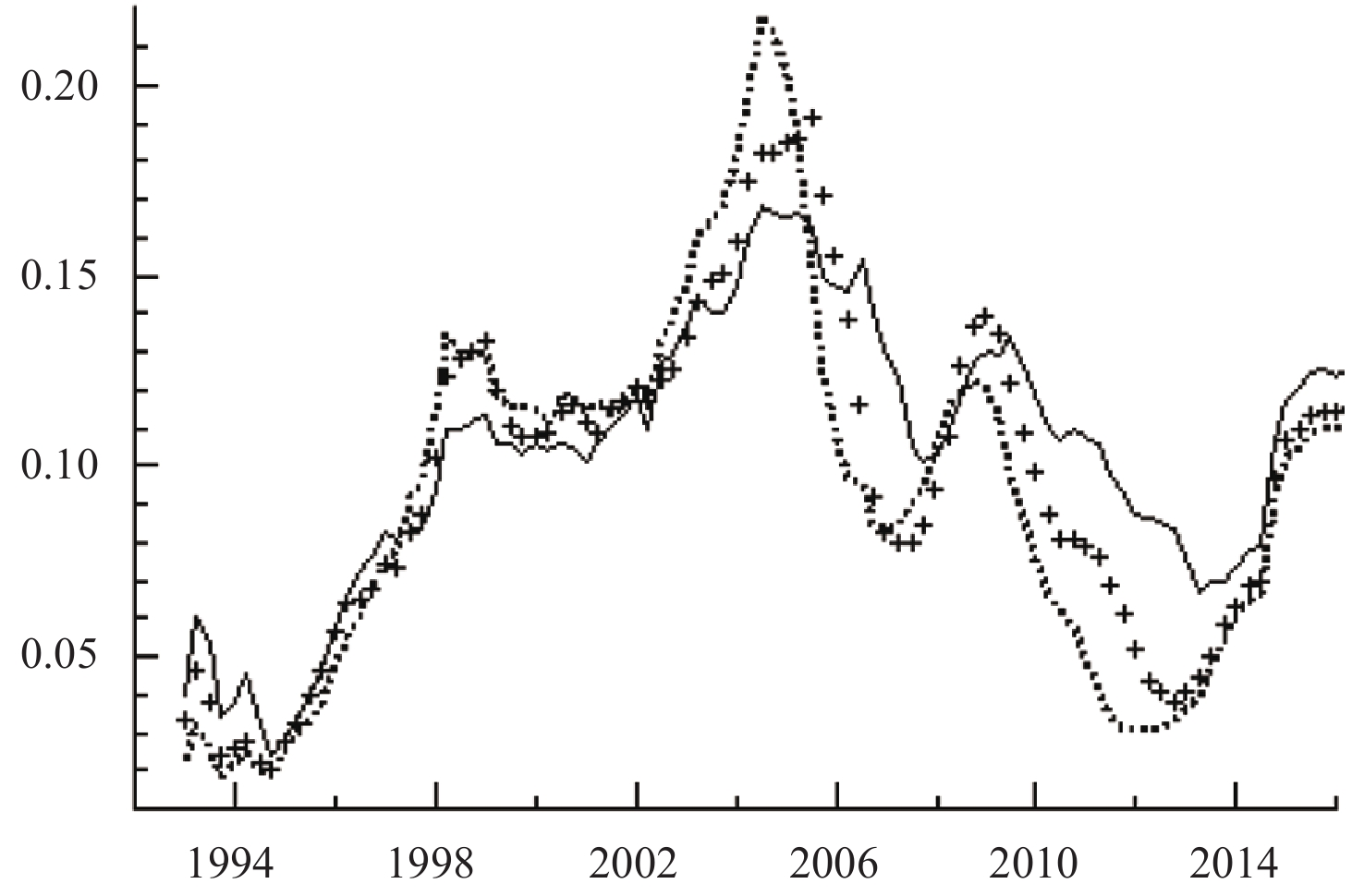图 4 金融效率对经济增长冲击的时变响应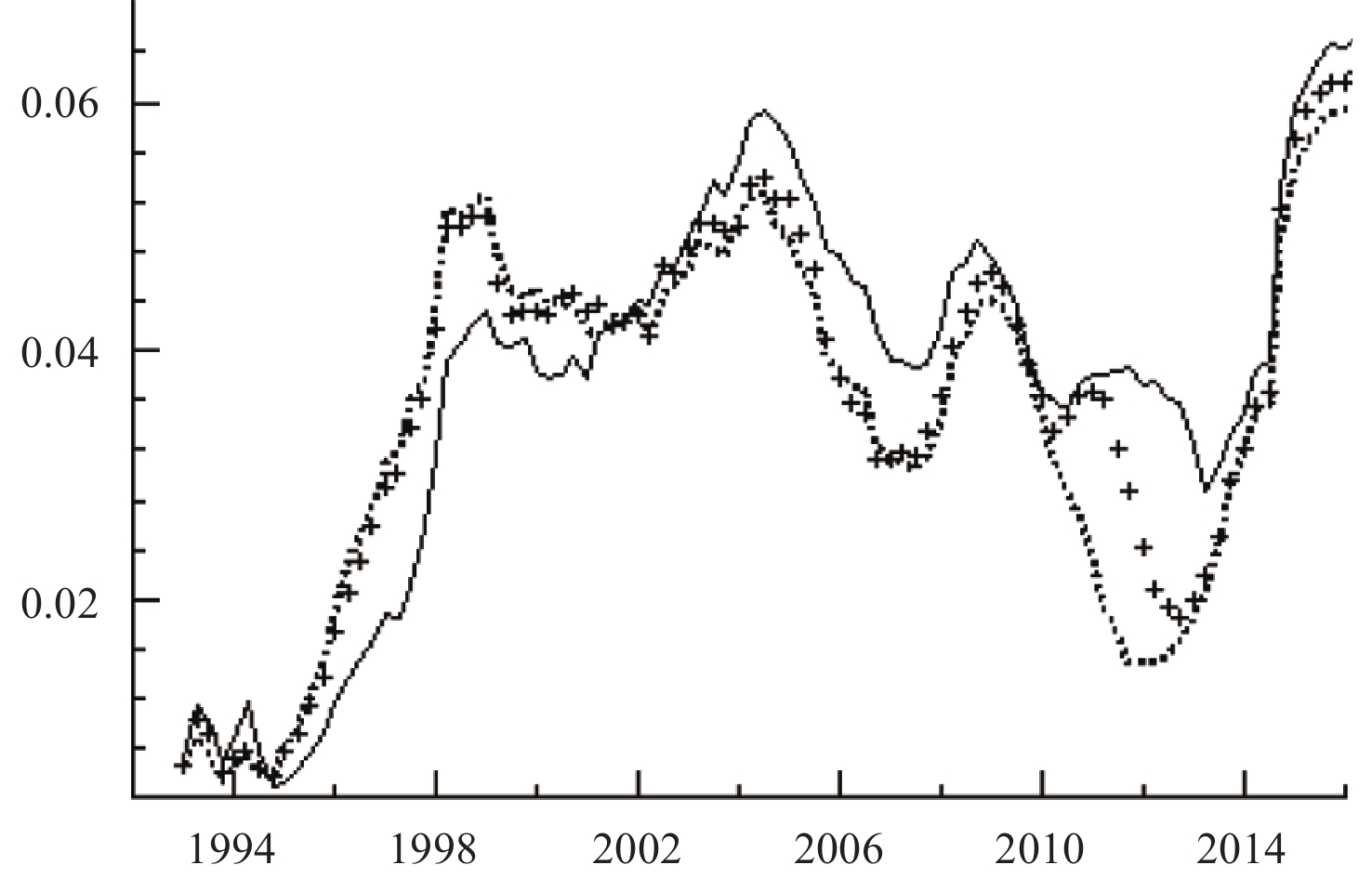图 5 金融效率对技术进步冲击的时变响应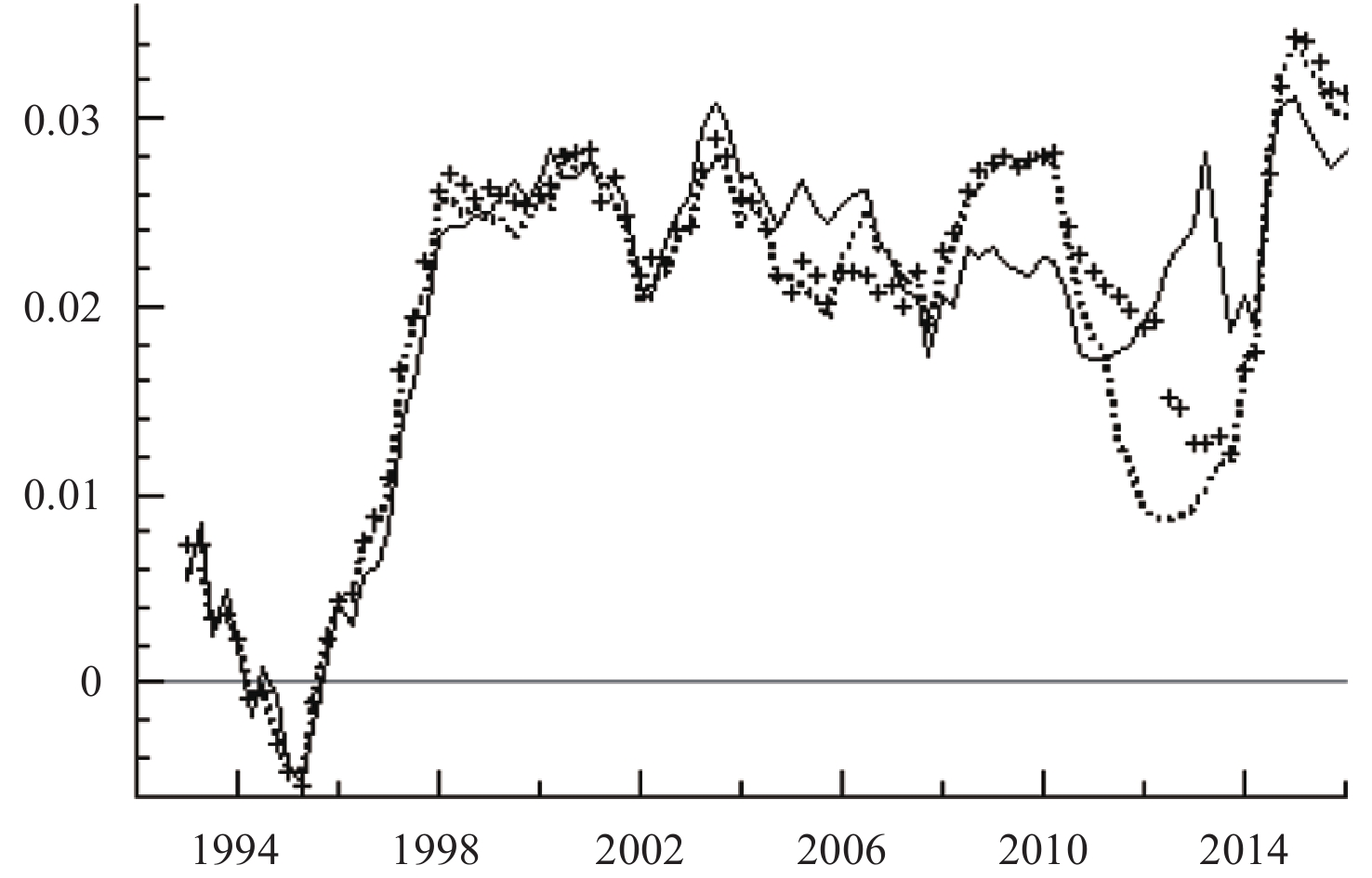图 6 技术进步对金融效率冲击的时变响应

“中等收入陷阱”问题是我国正在面临的一个顶级真问题，而鲜有文献从收敛性的角度对我国的中等收入陷阱问题进行研究。本文通过门限回归模型的建立，对我国经济增长的收敛路径进行识别与检验，研究发现，我国目前的经济增长正处于收敛态势，且这一收敛曲线的稳态值为负，说明现有收敛曲线无法使我国步入高收入阶段，我国只有转变经济增长的驱动因素，才有可能顺利成为高收入国家。为此，本文借助LT-TVP-VAR模型，研究“双轮驱动”因素对经济增长影响的动态特征，以此对我国是否具备向高收入阶段跃升的动力条件进行判断，通过对脉冲响应函数进行分析，可以得到以下结论：第一，技术进步通过规模效应、集聚效应以及溢出效应对经济增长表现出明显的正向作用，这一作用与前文识别的收敛路径高度耦合，在经济新常态时期，由于创新驱动发展战略的有效实施，这一促进作用较之前有明显的增强；第二，金融机构通过提高其资源配置效率对经济增长产生显著的积极作用，但随着金融市场业务的扩大，其虚拟投资对实体投资的挤占则会引致一定的金融风险，不利于经济的增长，我国金融效率对经济增长的影响整体上表现为正向效应，但这一正向作用从2005年起就逐渐减弱，至金融危机爆发降至最低，而经济新常态以来，随着供给侧改革的开展，我国在优化投资结构，提高资源配置效率等方面取得了相当的进展，金融效率对经济增长的促进作用得到了显著提升；第三，就技术进步与金融效率间的相互作用而言，二者之间以相互强化的方式发挥其内生作用。具备高风险、高投入以及更新速度快特点的创新活动离不开高效率的金融支持，这要求金融市场充分发挥其风险分散、资金配置以及价值发现的功能，以促进科技成果转化为生产力。而技术进步反过来也能推动科技创新，改变传统的运营模式、对象及产品，促进金融效率的提高，进而更好地服务创新活动与实体经济，二者相互推动，缺一不可。但金融效率对科技进步的促进作用要强于科技进步对金融效率的促进作用，表现出非对称性特征。经济新常态以来这两种促进作用的上升幅度明显提升，说明“双轮驱动”因素间正在以前所未有的水平相互融合，经济增长动力转换正在有效进行。

  蔡昉. " 中等收入陷阱”的理论、经验与针对性[J].经济学动态,2011(12).  陈守东, 孙彦林, 毛志方. 新常态下中国经济增长动力的阶段转换研究[J].西安交通大学学报(社会科学版),2017(1).  段世德, 徐璇. 科技金融支撑战略性新兴产业发展研究[J].科技进步与对策,2011(14).  贾康, 苏京春. 中国的坎: 如何跨越" 中等收入陷阱”[M]. 北京: 中信出版社, 2016.  林志帆. " 中等收入陷阱”存在吗?——基于增长收敛模型的识别方法[J].世界经济研究,2014(11).  刘伟. 突破" 中等收入陷阱”的关键在于转变发展方式[J].上海行政学院学报,2011(1).  马岩. 我国面对中等收入陷阱的挑战及对策[J].经济学动态,2009(7).  [英国]约翰•希克斯. 经济史理论[M].北京: 商务印书馆, 1987.  [美国]约瑟夫•熊彼特. 经济发展理论[M]. 北京: 商务印书馆, 1990.  闫森. 经济增长收敛与" 中等收入陷阱”——基于亚洲经济体的实证研究[J].亚太经济,2017(1).  赵昌文, 陈春发, 唐英凯. 科技金融[M]. 北京: 科学出版社, 2009.  Acemoglu D. Introduction to modern economic growth[M]. Princeton: Princeton University Press, 2008.  Aghion P, Howitt P. The economics of growth[M]. Cambridge: MIT Press, 2008.  Aiyar S S, Duval R, Puy D, et al. Growth slowdowns and the middle-income trap[R]. Washington: International Monetary Fund, 2013.  Barro R J, Salai-Martin X. Convergence[J].Journal of Political Economy,1992,100(2):223–251.  Eichengreen B, Park D, Shin K. When Fast-Growing economies slow down: International evidence and implications for China[J].Asian Economic Papers,2012,11(1):42–87.  Eichengreen B, Park D, Shin K. Growth slowdowns redux: New evidence on the middle-income trap[R]. NBER Working Paper No. w18673, 2013.  Hansen B E. Inference when a nuisance parameter is not identified under the null hypothesis[J].Econometrica,1996,64(2):413–430.  Hausmann R, Pritchett L, Rodrik D. Growth accelerations[J].Journal of Economic Growth,2005,10(4):303–329.  Ito T. Growth convergence and the Middle-income trap[J].Asian Development Review,2017,34(1):1–27.  Kharas H, Kohli H. What is the middle income trap, why do countries fall into it, and how can it be avoided?[J].Global Journal of Emerging Market Economies,2011,3(3):281–289.  Lucas R E Jr. On the mechanics of economic development[J].Journal of Monetary Economics,1988,22(1):3–42.  Nakajima J, West M. Bayesian analysis of latent threshold dynamic models[J]. Journal of Business & Economic Statistics,2013,31(2):151–164.  Ohno K. Avoiding the middle-income trap: Renovating industrial policy formulation in Vietnam[J].ASEAN Economic Bulletin,2009,26(1):25–43.  Primiceri G E. Time varying structural vector autoregressions and monetary policy[J].The Review of Economic Studies,2005,72(3):821–852.  Schwartz E S, Moon M. Evaluating research and development investments[A]. Brennan M J, Trigeorgis L. Project flexibility, agency, and competition: New development in the theory and application of real options[M]. New York: Dxford University Press, 2000.  Solow R M. A contribution to the theory of economic growth[J].The Quarterly Journal of Economics,1956,70(1):65–94.
The Path Breakthrough of Escaping the “Middle-income Trap” in China: Based on an Identification Method of Growth Convergence Theory and “Two-wheel Drive” Test
Liu Jinquan, Wang Qiaoru, Liu Dayu
Center for Quantitative Economics, Jilin University, Jilin Changchun 130012, China
Summary: The " middle-income trap” is a primary problem for China because it is at the stage of middle income level. The essence of this problem can be attributed to the convergence of economic growth. In other words, the " middle-income trap” can be understood as a relatively stable state in which a country converges at the middle-income level. Then, does the decline in potential growth in the new normal period mean that our country is converging to the middle-income level? This proposition can re-examine the changes in speed, structure and momentum in the new normal period from the perspective of " middle-income trap”, and then provide a new theoretical thought for cognizing and leading the new normal. For this reason, this paper demonstrates the convergence path of China’s economic growth through the theory of economic growth convergence. The results show that China’s current economic growth is in the convergence curve of middle income, and it is difficult to successfully converge to the high-income stage along the existing curve. Further, it is not enough to discuss whether there is a trap, what is more important is how to overcome this trap and connect the " Chinese dream”. As the replacement of traditional economic drivers during the strategic transition period, the two major drivers of science & technology and finance, namely, " two-wheel drive”, have become two important engines for China’s innovation-driven development. These two factors will be the key to our success in crossing the middle-income trap. For this reason, the LT-TVP-VAR model is used to study the dynamic influence of the two driving factors on the economic growth, so as to judge whether China has the essential momentum to jump to the high income stage. The results show that the promotion roles of technological progress and financial efficiency in economic growth are highly coincided with the convergence path identified in this paper, and they have been improved obviously in the new normal period. The two driving factors play the endogenous role in a mutually reinforcing way and have a higher level of integration in the new normal period, which indicate that the staggered transformation of momentum in China has taken effect. Therefore, in order to successfully jump to high income levels and achieve " catching-up” strategy, we need to promote the in-depth integration of technology and finance through governments and market jointly, and explore the development potential of the two major factors.
Key words: middle-income trap     economic growth convergence     two-wheel drive